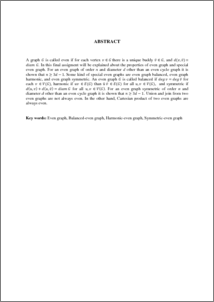# GRAF GENAP

SARI, VIANITA and Sumanto, Y.D. and IRAWANTO, BAMBANG (2010) GRAF GENAP. Undergraduate thesis, Fakultas Matematika dan Ilmu Pengetahuan Alam.Preview
PDF
33Kb

## Abstract

A graph G is called even if for each vertex v∈G there is a unique buddy v ̅∈G, and d(v,v ̅ ) = diam G. In this final assigment will be explained about the properties of even graph and special even graph. For an even graph of order n and diameter d other than an even cycle graph it is shown that n≥3d-1. Some kind of special even graphs are even graph balanced, even graph harmonic, and even graph symmetric. An even graph G is called balanced if deg⁡〖v=deg⁡v ̅ 〗 for each v ∈V(G), harmonic if uv ∈E(G) than u ̅ v ̅ ∈E(G) for all u,v ∈V(G), and symmetric if d(u,v)+d(u,v ̅ )=diam G for all u,v ∈V(G). For an even graph symmetric of order n and diameter d other than an even cycle graph it is shown that n≥3d-1. Union and join from two even graphs are not always even. In the other hand, Cartesian product of two even graphs are always even.

Item Type: Thesis (Undergraduate) Q Science > QA Mathematics Faculty of Science and Mathematics > Department of Mathematics 26891 Ms Niken P 20 Apr 2011 08:53 20 Apr 2011 08:53

Repository Staff Only: item control page